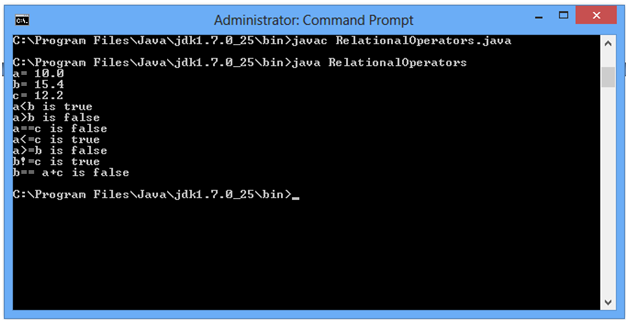## Relational operators in Java

Relational operators in Java
class RelationalOperators
{
public static void main(String args[])
{
float a=10.0F,b=15.4F,c=12.2F;
System.out.println("a= " +a);
System.out.println("b= " +b);
System.out.println("c= " +c);
System.out.println("a<b is " +(a<b));
System.out.println("a>b is " +(a>b));
System.out.println("a==c is " +(a==c));
System.out.println("a<=c is " +(a<=c));
System.out.println("a>=b is " +(a>=b));
System.out.println("b!=c is " +(b!=c));
System.out.println("b== a+c is " +(b==a+c));
}
}
OUTPUT